Next: Simplification procedure Up: General purpose solving algorithm Previous: Solutions and Distinct solutions   Contents

The 3B method

In addition to the classical bisection process all the solving algorithms in the ALIAS library may make use of another method called the 3B-consistency approach .

Although its principle is fairly simple it is usually very efficient (but not always, see section 2.4.3.1). In this method we consider each variable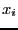in turn and its range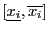. Let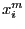be the middle point of this range. We will first calculate the interval evaluation of the functions in the system with the full ranges for the variable except for the variable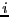where the range will be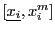. Clearly if one of the equations is not satisfied (i.e. its interval evaluation does not contain 0), then we may reduce the range of the variableto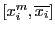. If this is not the case we will define a newas the middle point of the intervaland repeat the process until either we have found an equation that is not satisfied (in which case the interval for the variablewill be reduced to) or the width of the intervalis lower than a given threshold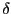. Using this process we will reduce the range for the variableon the left side and we may clearly use a similar procedure to reduce it on the left side. The 3B procedure will be repeated if:

• the variable ALIAS_Full3B is set to 1 or 2 (default value: 0) and if there are two changes on the variable (a change is counted when a variable is changed either on the left or right side) or the change in at least one variable is larger than ALIAS_Full3B_Change
• the variable ALIAS_Full3B is set to 1 and the change in at least one variable is larger than
ALIAS_Full3B_Change

For all the algorithms of ALIAS this method may be used by setting the flag ALIAS_Use3B to 1 or 2. In addition you will have to indicate for each variable a thresholdand a maximal width for the range (if the width of the range is greater than this maximal value the method is not used). This is done through the VECTOR variables ALIAS_Delta3B and ALIAS_Max3B. The difference of behavior of the method if ALIAS_Use3B is set to 1 or 2 is the following:

• 1: let e be the value of ALIAS_Delta3B for the current variable which is in the range [a,b]. On the left side we will check if [a,a+e] may lead to no solution. If yes then the current value of the variable is [a+e,b]. We will start again but this time we will double the size of of the interval we will check i.e. we will test the elimination of [a+e,a+3e], then [a+3e,a+7e] and will stop as soon as the check on one interval fail. For example assume that the test for [a+3e,a+7e] fails, then the updated range for the variable will be [a+3e,b].
• 2: the procedure at the beginning is similar to the previous one but changes when the check fails. In the previous example after the failure for [a+3e,a+7e] we will start again to examine if interval with width e can be eliminated. Hence we will check [a+3e,a+4e], then [a+4e,a+6e] and so on. In consequence in this mode we will get as left bound for the interval the highest possible value A such that [A,A+e] cannot be eliminated. Clearly in that case the procedure will be more computer intensive but will produce better results.
A typical example for a problem with 25 unknowns will be:

ALIAS_Use3B=1;
Resize(ALIAS_Delta3B,25);Resize(ALIAS_Max3B,25);
for(i=1;i<=25;i++)
{
ALIAS_Delta3B(i)=0.1;ALIAS_Max3B(i)=7;
}
which indicate that we will start using the 3B method as soon as the width of a range is lower than 7 and will stop it if we cannot improve the range by less than 0.1.

A drawback of the 3B method is that it may imply a large number of calls to the evaluation of the functions. The larger number of evaluation will be obtained by setting the ALIAS_Use3B to 2 and ALIAS_Full3B to 1 while the lowest number will be obtained if these values are 1 and 0. It is possible to specify that only a subset of the functions (the simplest) will be checked in the process. This is done with the global variable ALIAS_SubEq3B, an integer array whose size should be set to the number of functions and for which a value of 1 at positionindicates that the functionwill be used in the 3B process while a value of 0 indicates that the function will not be used. For example:

Resize(ALIAS_SubEq3B,10);
Clear(ALIAS_SubEq3B);
ALIAS_SubEq3B(1)=1;
ALIAS_SubEq3B(2)=1;
indicates that only the two first functions will be used in the 3B process. If you are using your own solving procedure, then it is necessary to indicate that only part of the equations are used by setting the flag ALIAS_Use_SubEq3B to 1.

In some cases it may be interesting to try to use at least once the 3B method even if the width of the range is larger than ALIAS_Max3B. If the flag ALIAS_Always3B is set to 1, then the 3B will be used once to try to remove the left or right half interval of the variables.

If you are using also a simplification procedure (see section 2.3.3) you may avoid using this simplification procedure by setting the flag ALIAS_Use_Simp_In_3B to 0. You may also adapt the simplification procedure when it is called within the 3B method. For that purpose the flag ALIAS_Simp_3B is set to 1 instead of 0 when the simplification procedure is called within the 3B method. For some procedure if ALIAS_Use_Simp_In_3B is set to 2 then ALIAS_Simp_3B is set to 1 when the whole input is checked. But if ALIAS_Use_Simp_3B is set to a value larger than 2 then ALIAS_Simp_3B is set to 0.

Some methods allows to start the 3B method not by a small increment that is progressively increased but by a large increment (half the width of the interval) and to decrease it if it does not work. This is done by setting the flag ALIAS_Switch_3B to a value between 0 and 1: if the width of the current interval is lower than the width of the initial search domain multiplied by this flag, then a small increment is used otherwise a large increment is used.

When the routine that evaluate the expression uses the derivatives of the expression we may avoid to use these derivatives if the width of the ranges in the box are too large. This is obtained by assigning the size of the vector ALIAS_Func_Grad to the number of unknowns and assigning to the components of this vector to the maximal width for the ranges of the variables over which the derivatives will not be used: if there is a range with a width larger than its limits then no derivatives will be used.

Note also that the 3B-consistency is not the only one that can be used: see for example the ALIAS-Maple manual that implements another consistency test for equations which is called the 2B-consistency or Hull-consistency in the procedure HullConsistency (similarly HullIConsistency implement it for inequalities). See also the section 2.17 for an ALIAS-C++ implementation of the 2B and section 11.4 for detailed calls to the 3B procedures.Next: Simplification procedure Up: General purpose solving algorithm Previous: Solutions and Distinct solutions   Contents
Jean-Pierre Merlet 2012-12-20